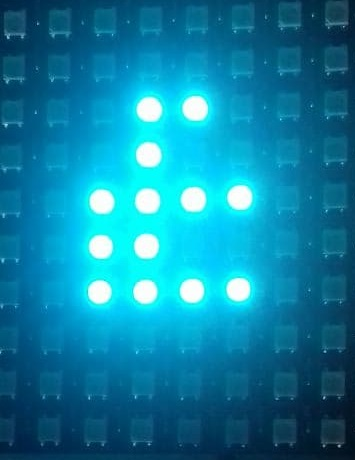# Matrix X,Y plotting with the Neopixel service

Python code to enable x,y plotting from the neopixel service, an inviting rabbit hole.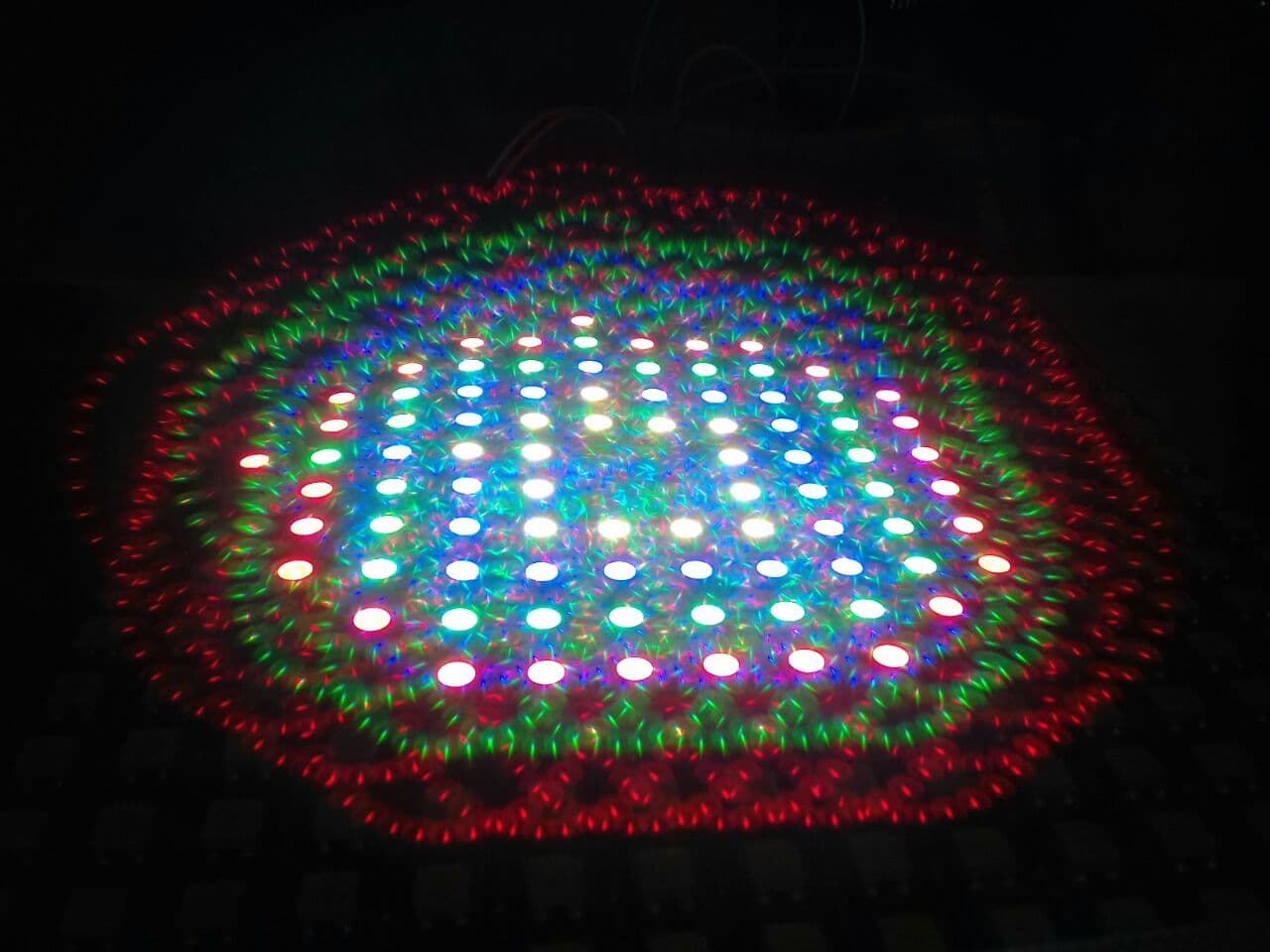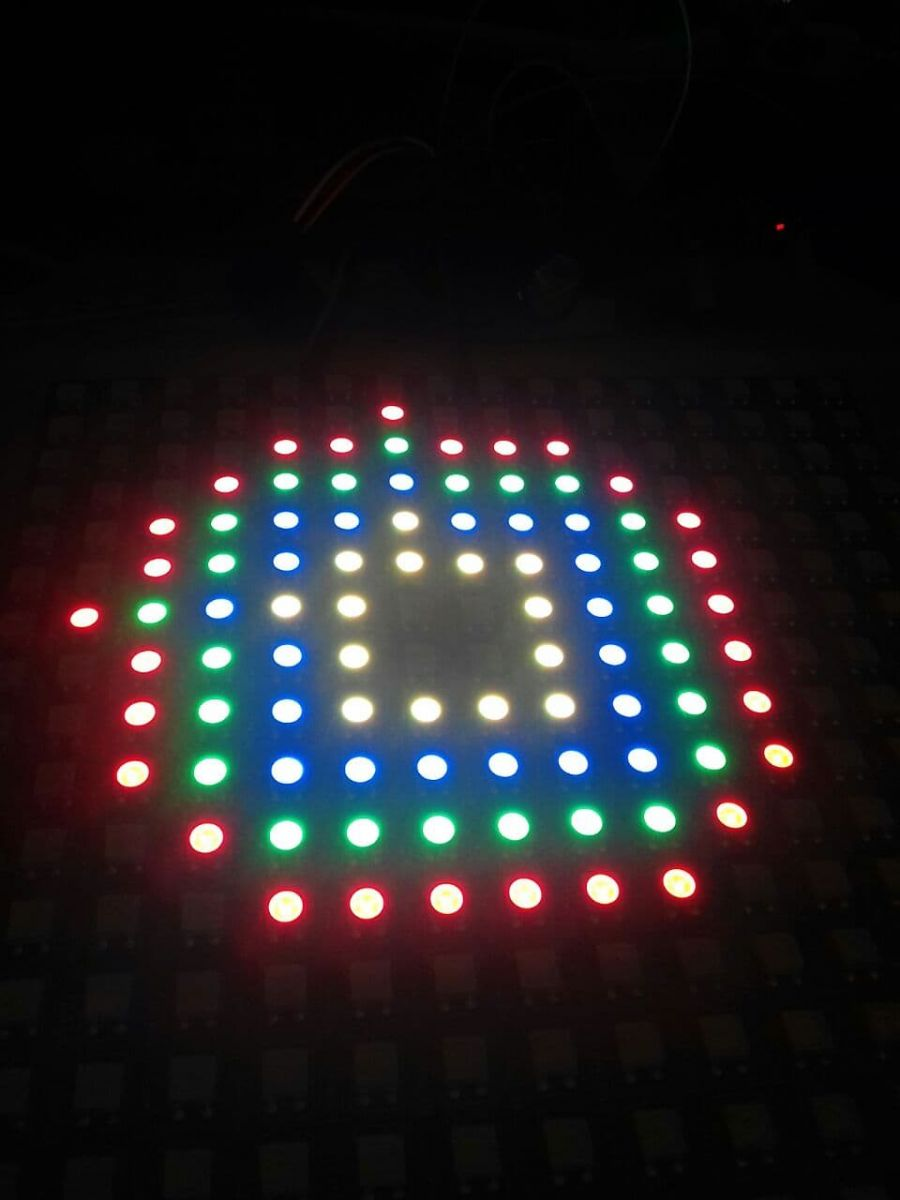Many Matrix displays have a serpentine LED pattern. This means that odd and even columns are reversed.

The code below unravels the switching of the columns, all you have to do is feed the x and y coords in and the code will determine the pixel that needs to be displayed. The highlighted text indicates this conversion.

Usage - Call the definition :- def XYtoPixel(x,y,r,g,b): #supply x,y, and rgb colour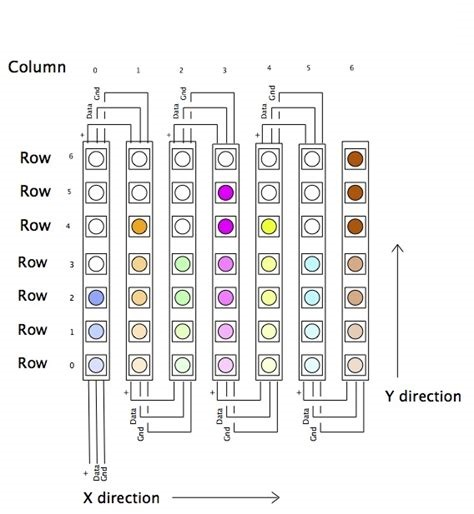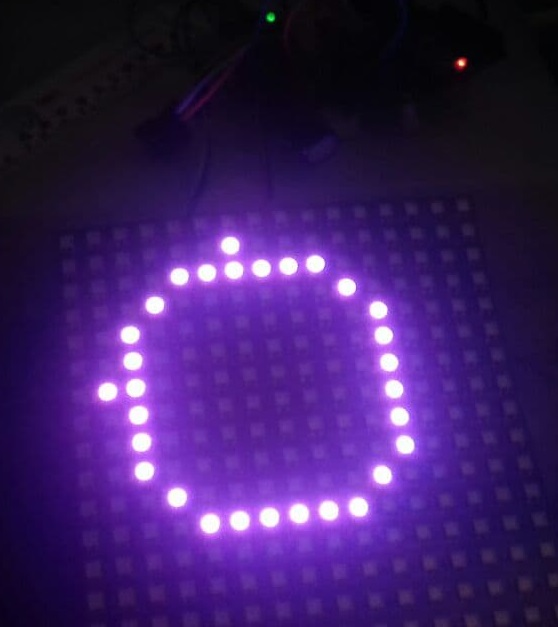Python sketch for a 16*16 Neopixel display import math port = "COM15" pin = 3 pixelCount = 216 # starting arduino arduino = Runtime.start("arduino","Arduino") arduino.connect(port) # starting neopixle neopixel = Runtime.start("neopixel","NeoPixel") neopixel.setPin(pin) neopixel.setPixelCount(pixelCount) # attach the two services neopixel.attach(arduino) def XYCircle(radiusx,radiusy,r,g,b):    #Test code for drawing circle  for pie in range(0,359,6):   y=9+(radiusy*(math.cos(math.radians(pie))))   x=9+(radiusx*(math.sin(math.radians(pie))))   XYtoPixel(x,y,r,g,b)   #print(i),(x),(y) def XYtoPixel(x,y,r,g,b): #supply x,y, and rgb colour  x=int(x)  y=int(y)  z = x & 1       #Test odd or even column  if  z == 0 :     #Even column    pixel = 16 * (16 - (x+1))+y  else :            #Odd column    pixel = 16 * (16 - x) - (y+1)    neopixel.setPixel(pixel,r,g,b)   XYCircle(6,6,255,0,255)  #Plot the circles  expects x and y radii + RGB colours XYCircle(5,5,255,255,0) XYCircle(4,4,0,255,255) XYCircle(3,3,255,255,255)

With x,y plotting, its also possible to place and add text/numbers (maybe as debug tool - for displaying startup messages)

Coming soon :- (erhmmmm with reversal)